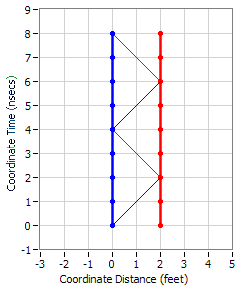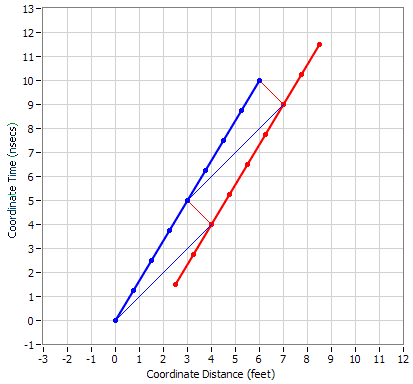# Light clock on Train experiment.

In the moving frame, relative to the stationary frame, if time is slowed and the speed of light isn't, why doesn't the light travel further in less elapsed time?

•DaveC426913
Gold Member
You need to define your points of view. Where does the light travel? On the train? Next to the train?

Nugatory
Mentor
In the moving frame, relative to the stationary frame, if time is slowed and the speed of light isn't, why doesn't the light travel further in less elapsed time?

You always have to consider three effects:
- relativity of simultaneity
- time dilation
- length contraction
The way you've asked your question, you are only considering time dilation.

One good exercise is drawing a space-time diagram of the exact situation that you are considering with the paths of both observers, the light emission event, the light detection event, and the path of the light all shown. Do that, and you'll likely see how it all works consistently.

Another good exercise is to write down the the x and t coordinates of all the relevant events:
- emission event
- absorption event
- position of emitter at time of absorption according to train observer
- position of emitter at time of absorption according to platform observer
- position of receiver at time of emission according to train observer
- position of receiver at time of emission according to platform observer
in one or the other frame, and then use the Lorentz transforms (not the time dilation and length contraction formulas!) to transform into the other frame.

You need to define your points of view. Where does the light travel? On the train? Next to the train?
The light clock is on the train as in the standard thought experiment.
You always have to consider three effects:
- relativity of simultaneity
- time dilation
- length contraction
The way you've asked your question, you are only considering time dilation.

One good exercise is drawing a space-time diagram of the exact situation that you are considering with the paths of both observers, the light emission event, the light detection event, and the path of the light all shown. Do that, and you'll likely see how it all works consistently.

Another good exercise is to write down the the x and t coordinates of all the relevant events:
- emission event
- absorption event
- position of emitter at time of absorption according to train observer
- position of emitter at time of absorption according to platform observer
- position of receiver at time of emission according to train observer
- position of receiver at time of emission according to platform observer
in one or the other frame, and then use the Lorentz transforms (not the time dilation and length contraction formulas!) to transform into the other frame.

The light clock-train thought experiment is about time dilation therefore so is my question. I don't have a physics background. Perhaps you could provide a simpler answer
i.e why doesn't light travel further in less elapsed time.

Nugatory
Mentor
The light clock-train thought experiment is about time dilation therefore so is my question. I don't have a physics background. Perhaps you could provide a simpler answer
i.e why doesn't light travel further in less elapsed time.

Using either frame when you divide the distance the light travels by the time it travels, you have to get ##c##.

When the light clock is oriented perpendicular to the direction of motion and the arm is of length ##L##, the distance travelled in the train frame is ##2L##, so the time measured by the train observer (at rest relative to the clock) is ##T_t=2L/c##. The platform observer (moving relative to the train) sees the slantwise distance travelled to be ##2\gamma{L}## and therefore measures the time for one round-trip tick to be ##T_p=2\gamma{L}/c##. ##\gamma## is greater than one so less train time passes during one tick and the light travels a shorter distance in the train frame.

(You can calculate that ##2\gamma{L}## above from the Pythagorean theorem. The length of the slantwise path is ##\sqrt{L^2+(vT_p)^2}## and the time for the light to travel out and back on that path has to be equal to ##2T_p##. A bit of algebra will see you home from there).

Last edited:
ghwellsjr
Gold Member
The light clock-train thought experiment is about time dilation therefore so is my question. I don't have a physics background. Perhaps you could provide a simpler answer
i.e why doesn't light travel further in less elapsed time.
Why do you say "less elapsed time"? Time Dilation means it takes more elapsed Coordinate Time for the same amount of Proper Time. Sometimes we say the moving clock is ticking more slowly in the frame in which it is moving but that just means it takes longer for it to tick in the moving frame than it does in its rest frame.

To illustrate let's look first at a spacetime diagram for a light clock in its rest frame. The worldlines of the two mirrors are indicated by the thick blue and red lines while the light reflecting back and forth between them are indicated by the thin lines. The light starts at the bottom.Note that it takes 4 nanoseconds of Coordinate Time for the light clock to go through one cycle as indicated by the grid lines and the graph markings. It also takes 4 nanoseconds of Proper Time as indicated by the blue dots which are spaced one nanosecond apart. Note that the speed of light is one foot per nanosecond and appears as 45-degree diagonal lines. Since the mirrors are 2 feet apart, the light travels a total 4 feet in a total of 4 nanoseconds per cycle of the light clock.

So far so good? OK, now let's transform to the rest frame of the ground where the train with its light clock are traveling at 0.6c:Now you can see that it takes 5 nanoseconds of Coordinate Time for the light clock to go through one cycle and the light has to travel 4 feet of Coordinate Distance in one direction and 1 foot back for a total of 5 feet of Coordinate Distance. Note that the blue dots marking off the Proper time for the light clock are spaced out so that 4 nanoseconds of Proper Time takes 5 nanoseconds of Coordinate Time. That's what Time Dilation is--the ratio of Coordinate Time to Proper Time.

While we're at it, you can also see the Length Contraction of the spacing between the mirrors being reduced from 2 feet in their rest frame to 1.6 feet of Coordinate Distance in the frame in which they are moving at 0.6c. The Lorentz factor at this speed is 1.25

and if less time is elapsed, does the light travel further?

ghwellsjr
Gold Member
and if less time is elapsed, does the light travel further?
According to the second postulate of Special Relativity, light always travels at c according to the coordinates of any Inertial Reference Frame. It takes more Coordinate Time to travel a greater Coordinate Distance. Isn't this clear in the diagrams? Where do you see "less time elapsed"?

Time passes more slowly in the moving frame. One second becomes o n e s e c o n d, therefore less time elapses. The light has more time to travel the longer mirror to mirror distance, whilst always travelling at c.

Nugatory
Mentor
Time passes more slowly in the moving frame. One second becomes o n e s e c o n d, therefore less time elapses. The light has more time to travel the longer mirror to mirror distance, whilst always travelling at c.

One second is defined to be the time that it takes for light to travel a distance of 299792458 meters, so it is not possible for the light to travel any other distance in one second.

Let's say that you, at rest relative to the light clock, observe that the light travels 299792458 meters to the mirror and then the same distance back - your wristwatch ticks twice.

I, moving relative to you and the light clock (or you and the light clock are moving relative to me - it's the same thing) observe that the light travels on the slantwise path out and back, so covers a longer distance during its out and back trip. My wristwatch ticks more than twice, so the light has more time and travels farther using my frame rather than yours - but as my wristwatch ticked more than twice while yours ticked twice, I'll say that you're the one whose time is dilated.

(you will, of course, say exactly the same thing about my time).

The moving clock takes longer to tick over because the distance is greater, but it does so in slowed time. From the instant light has a diagonal path, time is slowed. The longer path is offset by time passing more slowly.

ghwellsjr
Gold Member
Time passes more slowly in the moving frame.
Let's make sure we agree on what this phrase means. It means: in the frame in which the clock is moving, the Proper Time of the clock passes more slowly than it does for the Coordinate Time of the frame, agreed?

One second becomes o n e s e c o n d, therefore less time elapses.
Let's also make sure we agree on what this phrase means: It means one second of Proper Time for the moving clock gets stretched out to take more than one second of Coordinate Time and therefore less Proper Time elapses for the moving clock than Coordinate Time, agree?

The light has more time to travel the longer mirror to mirror distance, whilst always travelling at c.
The light has more Coordinate Time to travel the longer mirror to mirror Coordinateror Distance, whilst always traveling at c according to he, agree?